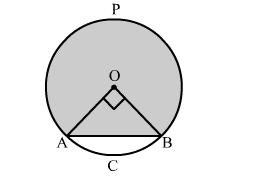# The radius of a circle with centre O is 7 cm.`
Question:

The radius of a circle with centre O is 7 cm. Two radii OA and OB are drawn at right angles to each other. Find the areas of minor and major
segments.

Solution:Area of minor segment = Area of sector AOBC − Area of right triangle AOB

$=\frac{90^{\circ}}{360^{\circ}} \pi(\mathrm{OA})^{2}-\frac{1}{2} \times \mathrm{OA} \times \mathrm{OB}$

$=\frac{1}{4} \times \frac{22}{7} \times(7)^{2}-\frac{1}{2} \times 7 \times 7$

$=\frac{1}{4} \times \frac{22}{7} \times(7)^{2}-\frac{1}{2} \times 7 \times 7$

$=38.5-24.5$

$=14 \mathrm{~cm}^{2}$

Area of major segment APB = Area of circle − Area of minor segment

$=\pi(\mathrm{OA})^{2}-14$

$=\frac{22}{7} \times(7)^{2}-14$

$=154-14$

$=140 \mathrm{~cm}^{2}$

Hence, the area of major segment is 140 cm2 .